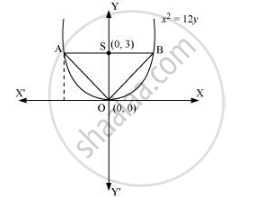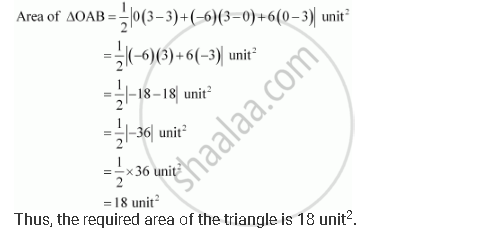CBSE (Arts) Class 11CBSE
Share
Notifications

View all notifications
Books Shortlist
Your shortlist is empty

# Find the Area of the Triangle Formed by the Lines Joining the Vertex of the Parabola X2 = 12y to the Ends of Its Latus Rectum. - CBSE (Arts) Class 11 - Mathematics

Login
Create free account

Forgot password?
ConceptParabola Latus Rectum

#### Question

Find the area of the triangle formed by the lines joining the vertex of the parabola x2 = 12y to the ends of its latus rectum.

#### Solution

The given parabola is x2 = 12y.

On comparing this equation with x2 = 4ay, we obtain 4a = 12 ⇒ a = 3

∴The coordinates of foci are S (0, a) = S (0, 3)

Let AB be the latus rectum of the given parabola.

The given parabola can be roughly drawn asAt y = 3, x2 = 12 (3) ⇒ x2 = 36 ⇒ x = ±6

∴The coordinates of A are (–6, 3), while the coordinates of B are (6, 3).

Therefore, the vertices of ΔOAB are O (0, 0), A (–6, 3), and B (6, 3).Is there an error in this question or solution?

#### APPEARS IN

Solution Find the Area of the Triangle Formed by the Lines Joining the Vertex of the Parabola X2 = 12y to the Ends of Its Latus Rectum. Concept: Parabola - Latus Rectum.
S